# The 80-lb block is attached to link AB and rests on a moving belt. Knowing that µs = 0.25 and µk = 0.20, determine the magnitude of the horizontal force P that should be applied to the belt to maintain its motion (a) to the right, (b) to the left.

Question-AnswerCategory: Engineering MechanicsThe 80-lb block is attached to link AB and rests on a moving belt. Knowing that µs = 0.25 and µk = 0.20, determine the magnitude of the horizontal force P that should be applied to the belt to maintain its motion (a) to the right, (b) to the left.

The 80-lb block is attached to link AB and rests on a moving belt. Knowing that µs = 0.25 and µk = 0.20, determine the magnitude of the horizontal force P that should be applied to the belt to maintain its motion (a) to the right, (b) to the left.Step: 1

Coefficient of static friction,Coefficient of kinetic friction,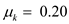Weight of the block,Step: 2

a)
Let us draw the free body diagram of the block considering the motion of the belt in the rightward direction.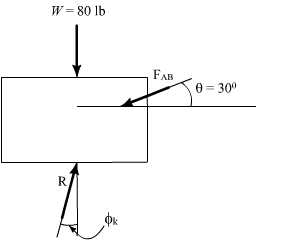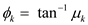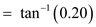Step: 3

Let us draw the force triangle.Step: 4

Apply law of sines and solve.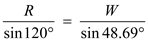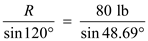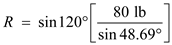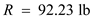Step: 5

Let us draw the free body diagram of the belt.Step: 6

Assume that the force in the positive x-direction is positive.From the equilibrium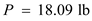Therefore, the magnitude of the horizontal force that should be applied to the belt to maintain its motion to the right is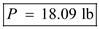b)
Let us draw the free body diagram of the block considering the motion of the belt in the leftward direction.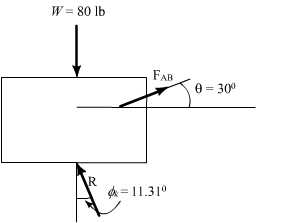Step: 7

Let us draw the force triangle.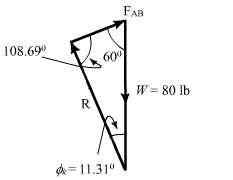Step: 8

Apply the law of sines and solve.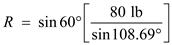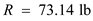Step: 9

Let us draw the free body diagram of the belt.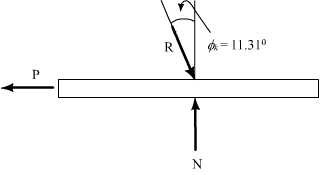Step: 10

Take the condition of equilibrium,and substitute the known values Assume that the force in the positive x-direction is positive.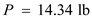Therefore, the magnitude of the horizontal force that should be applied to the belt to maintain its motion to the left is